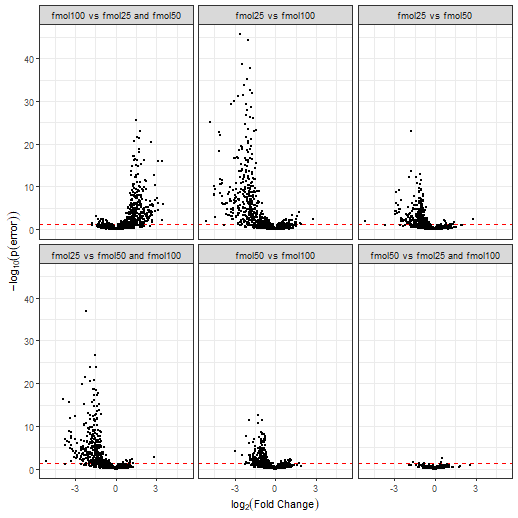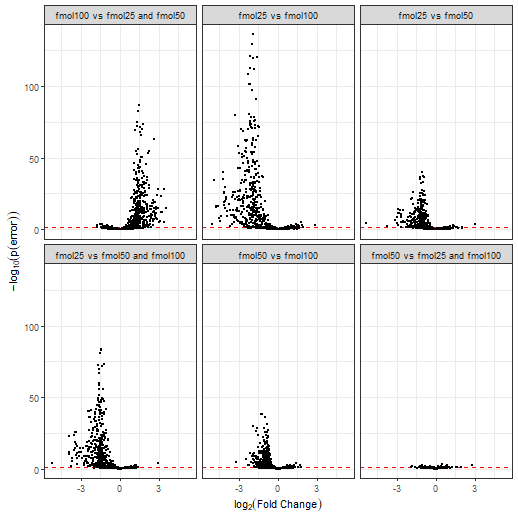# 1. Setup

Here we cover the tutorial of UPS-DS. The UPS-DS has one more condition than the yeast-DS and has one more replicate in each condition (yeast-DS is shown in vignette("baldur_yeast_tutorial")).

library(baldur)
# Packages for pre-processing
library(dplyr, warn.conflicts = FALSE)
library(tidyr, warn.conflicts = FALSE)

# Setup the design matrix
ups_design <- model.matrix(~ 0 + factor(rep(1:3, each = 4)))
colnames(ups_design) <- paste0('fmol', c(25, 50, 100))

# Set id column
id_col <- colnames(ups) # "identifier"

# Normalize and add M-V trend
ups_norm <- ups %>%
# Remove rows with NA for the sake of the tutorial
drop_na() %>%
# Normalize the data
psrn(id_col) %>%
calculate_mean_sd_trends(ups_design)

# For the contrast, we want to compare all conditions against each other, and in addtion, suppose we would like to know the difference between the mean of condition 1 and 3 against 2 ([fmol25 + fmol100]/2 - fmol50).
# This can easily be achieved with the following:
ups_contrast <- matrix(
c(
1,  1,   0,  1,   -0.5, -0.5,
-1,  0,   1, -0.5,  1,   -0.5,
0, -1,  -1, -0.5, -0.5,  1
), nrow = ncol(ups_design),
byrow = TRUE
)

Lets go over the design and contrast matrix. First, lets not how the design matrix uses subsets of the real columns of each condition:

colnames(ups_norm[-1])
#>   "fmol25_1"  "fmol25_2"  "fmol25_3"  "fmol25_4"  "fmol50_1"  "fmol50_2"  "fmol50_3"  "fmol50_4"  "fmol100_1" "fmol100_2"
#>  "fmol100_3" "fmol100_4" "mean"      "sd"
colnames(ups_design)
#>  "fmol25"  "fmol50"  "fmol100"

This lets baldur easily identify what columns that are of interest and setting up pre-cursors for the sampling. In addition, baldur gets information on the number of conditions there are in the data (i.e., ncol(ups_design)) and the number of replicates in each condition (i.e., colSums(ups_design)). Next, the contrast matrix:

ups_contrast
#>      [,1] [,2] [,3] [,4] [,5] [,6]
#> [1,]    1    1    0  1.0 -0.5 -0.5
#> [2,]   -1    0    1 -0.5  1.0 -0.5
#> [3,]    0   -1   -1 -0.5 -0.5  1.0

First, note how the contrast only has two columns; currently baldur only allows pair-wise comparisons. Lets go over the rows, the first row tells us that the first column of the design matrix should be compared against the second. I.e., the first row means fmol25 - fmol50. The second row shows that the first column should be compared against the third, i.e., fmol25 - fmol100. The third row shows that the second column should be compared against the third (fmol50 - fmol100). And, of course, the last row shows the mean of condition one and three vs condition two.

# 3. Sampling from the Data and Decision model

Finally we sample the posterior of each row in the data. baludr is very easy to run in parallel and this will drastically reduce the running time. The only thing that needs to be changed is the clusters flag:

# Single trend
gr_results <- gr %>%
estimate_gamma_hyperparameters(ups_norm, id_col) %>%
infer_data_and_decision_model(
id_col,
ups_design,
ups_contrast,
unc_gr,
clusters = 10 # Change this to 1 to run sequentially
)

Here err is the probability of error, i.e., the two tail-density supporting the null-hypothesis, lfc is the estimated log$$_2$$-fold change, sigma is the common variance, and lp is the log-posterior. Columns without suffix shows the mean estimate from the posterior, while the suffixes _025, _50, and _975, are the 2.5, 50.0, and 97.5, percentiles, respectively. The suffixes _eff and _rhat are the diagnostic variables returned by rstan (please see the Stan manual for details). In general, a larger _eff indicates a better sampling efficiency, and _rhat compares the mixing within chains against between the chains and should be smaller than 1.05. An important difference from yeast-DS is that each peptide gets three rows, one for each comparison in the contrast matrix.

# 4. Inference with Latent Gamma Mixuter Regression

To run Baldur with the LGMR model is very similar to running it with the GR model. First we fit the regression model using the fit_lgmr function. Here I will try to make use of my parallel processors to speed-up the inference:

ups_lgmr <- fit_lgmr(ups_norm, id_col, chains = 10, cores = 10, warmup = 1000, iter = 3000)

On rare occasions, the UPS-DS will have a few (<10) divergent transitions or exceed maximum tree depth, it is unlikely to have any impact on the final inference (considering that the posterior draws are 20 000). One could increase the acceptance rate and max tree depth at the expense of computational time. E.g., with:

ups_lgmr <- fit_lgmr(
ups_norm, id_col, chains = 10, cores = 10, warmup = 1000, iter = 3000,
control = list(adapt_delta = .95, max_treedepth = 11)
)

We can then estimate the uncertainties and hyperparameters for the data and decision model and run them as for the GR model:

# Estimate uncertainty
unc_lgmr <- estimate_uncertainty(ups_lgmr, ups_norm, id_col, ups_design)
# Sample from the data and decision model
lgmr_results <- ups_lgmr %>%
estimate_gamma_hyperparameters(ups_norm, id_col) %>%
infer_data_and_decision_model(
id_col,
ups_design,
ups_contrast,
unc_lgmr,
clusters = 10 # Change this to 1 to run sequentially
)

Plotting LGMR:

plot_lgmr_regression(ups_lgmr)
plot_regression_field(ups_lgmr, rng = 25)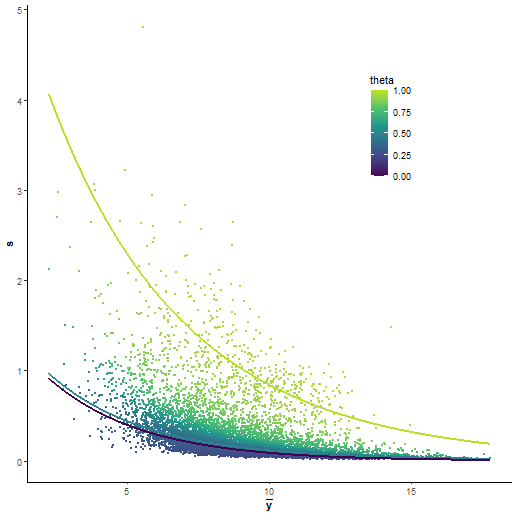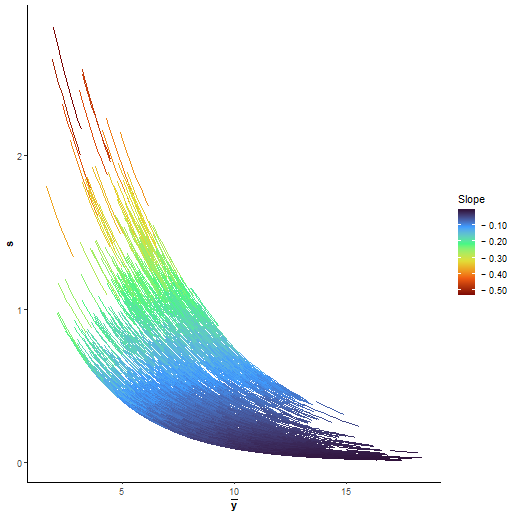# 5. Visualization of the results

baldur have two ways of visualizing the results 1) plotting sigma vs LFC and 2) Volcano plots. To plot sigma against LFC we use plot_sa:

gr_results %>%
plot_sa(
alpha = .05, # Level of significance
lfc = 1      # Add LFC lines
)

lgmr_results %>%
plot_sa(
alpha = .05, # Level of significance
lfc = 1      # Add LFC lines
)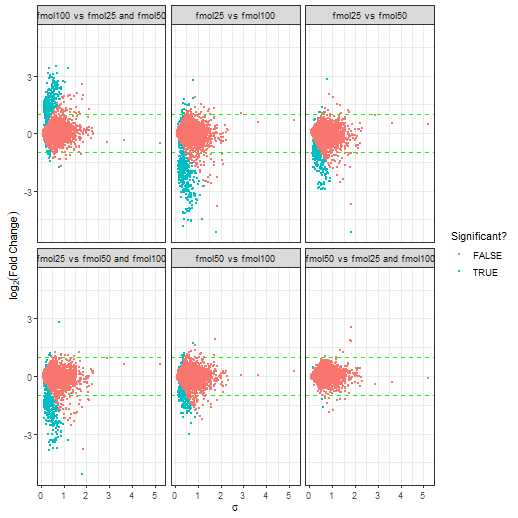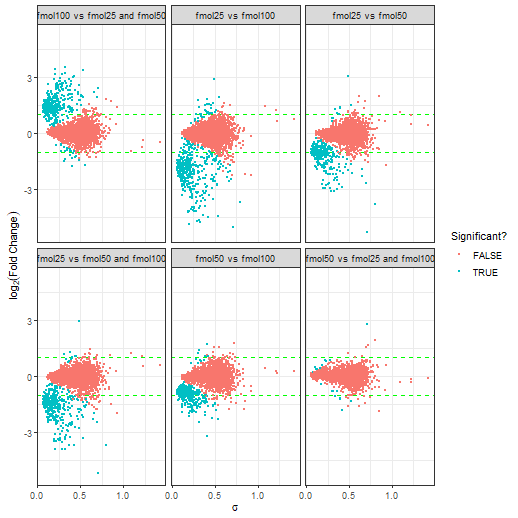In general, a good decision is indicated by a lack of a trend between $$\sigma$$ and LFC. We can see that Baludr with the LGMR model (second plot) has a lower trend compared to GR model (first plot) for which there is a trend for $$\sigma$$ to increase with LFC.

To make a volcano plot one uses plot_volcano in a similar fashion to plot_sa:

gr_results %>%
plot_volcano(
alpha = .05 # Level of significance
)

lgmr_results %>%
plot_volcano(
alpha = .05 # Level of significance
)